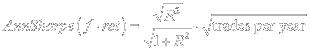## Monday, January 18, 2010

### R2s and Sharpes

In strategy simulations the performance metric is sometimes presented as an R2. These are difficult to interpret because clearly a 1% R2 over 1 day is a better strategy than one with an R2 of 1% over a month. A nice focal point is an annualized Sharpe ratio. Historically, the benchmark offered by the equity risk premium is a nice focal point, because it is common and feasible (around 0.4, as given a 6% equity premium, a 15% annualized vol (I wrote a book why I think this is probably more like 0.1, but that’s a minority view). Anything above 0.5 is quite good, and above 1.0 excellent. The annualized Sharpe ratio is define asBy using annualized Sharpes we can compare apples to apples. As returns are linear in time, standard deviation increases by the square root of time, so one needs to have a common time to make Sharpe ratio comparisons.

Now, for many strategies, one is predicting the profit on the combination of a specific tactical rule with a model that generates an expected return. For example, if I think the market is going up, one can take advantage of this several ways: buy futures, options, bull-call spreads, etc. This adds degrees of freedom to the result, because often certain criteria can generate different Sharpe ratios.

I will assume one implements a linear position rule, such that a position is linear in the expected return of the strategy. Thus, if one expects a return of 1%, one has half the long position as when one expects a return of 2%; if one expect a return of -1%, one has the opposite position as when the expected return is 1%. It is the proportionality between signals that affects the Sharpe ratio, not the absolute size, because whether I invest, on average, \$1k or \$1MM, it will have the same Sharpe ratio. As to whether linear is optimal, intuitively it is pretty close to optimal, basically saying that if conditional variance is constant, you put down twice as many chips as when you expect twice the return.

Thus, assume we are forecasting a return, which can be a portfolio, or a single stock, it does not matter. Some ‘thing’ has an expected return. This has a forecastable and nonforecastable component.Here f is the forecastable component, ε the random component, of the strategy return. Given the linear position rule, the exected return is simplyIt is useful to assume the unconditional expected return above the risk-free rate on the strategy and the forecast are both zero. To the extent they are not, that would be part of the predictable return that would not be ‘excess’, a cost of capital. Thus, with we haveNow, the variance of the product of two Gaussian random variables, applied to f and ret, is

Var(f⋅ret)=σ2f,ret+2σf,retE(f)E(ret)+E(f)2σ2ret+E(ret)2σ2f2fσ2ret

The proof for that is quite tedious (see Bohrnstedt and Goldberger, 1969), and crucially relies on the Gaussian distribution to avoid extra complexity from higher moments (that’s why we love the normal!).

Given the expected returns are both zero, we haveSo, the Sharpe of the strategy is justNow, given σx,yx,yσxσy we haveThe nice thing is that the numerator, ρx,y, is just the square root of an R2! That is, whatever your f, the R2 of a regression of the return on f, is what you need to know to calculated a Sharpe ratio.

The last step is to annualize, to put this into consistent units. To do this we simply apply the adjustment. For strategies that are not necessarily done over calendar time in constant increments, we assume t=number of sequential trades per year. This generates the following adjustment. You multiply the Sharpe by the square root of the expected number of trades per year. So, in this equation, you meake sure you have the number of expected trades in there, t.orSo, with an R2, and trading frequency, we can generate a reasonable Sharpe. For example, in the graph below, we see how the R2 of Sharpe 1.0 strategies vary by the number of trades made per year (eg, a horizon of 1 month trades 12 times a year).So, an R2 when forecasting annual data, is not nearly as valuable as the same R2 over 1 week. That's obvious on some level, but I think it's nice to have a specific formula to make such comparisons. An important qualifications is that transaction costs are not addressed here. The higher the frequency of the strategy, the higher the importance of transaction costs.

Unknown said...

Just a heads-up, the "white on transparent background" images are very hard to make out when viewed over a white background (for example, in Google Reader).

Charles Pennington said...

I think you made a mistake when you annualized.

I believe you should have simply multiplied the formula by sqrt(t). Instead, you put the radical around everything.

In other words, I think annualized Sharpe should be (rho/sqrt(1+rho^2))*sqrt(t).

Correct?

Thanks for your great blog.

Eric Falkenstein said...

egads, you're right! I spent so much time trying to get those darn equations to work I didn't check them enough. It seems, with MathType, the equations are buggy, and sometimes show up, sometimes not, as if there's a randomizer in them.